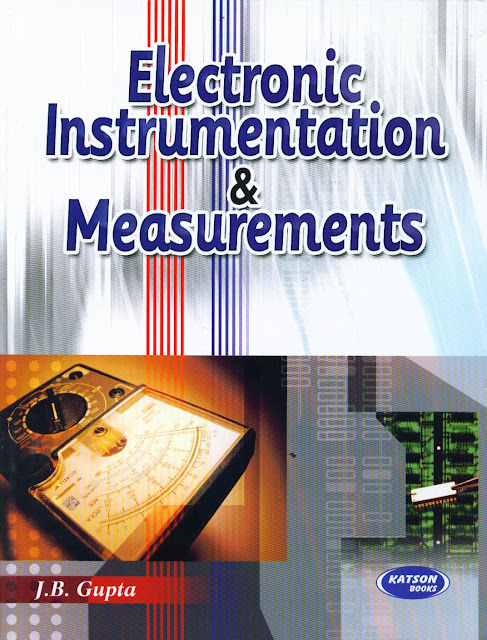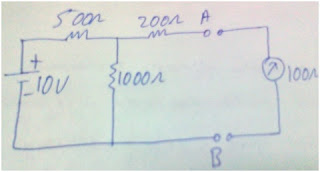### ECE 4th SEM IMP QUESTIONS

abhimanyu projects
August 28, 2012Posted by Abhimanyu

## Electronic Instrumentation and Measurements

Jan 01, 2013Posted by Abhi Raj

##Q.1. Calculate the maximum percentage error in the difference of two measured voltages when V1 = 200 V ± 2 % and V2 = 50 V ±6 %.

Q.2 Derive the equation for percentage error for  product of quantities of two or more quantities.

Q.3. Define accuracy, precision and resolution. How they are different from each other.

Q.4. Give applications of CRO. Why trigger circuit is used in CRO.

Q.5. A 0-300 V voltmeter has 600 scale divisions which can read to 1/2 division.   Determine the resolution of the meter in volt.

Q.6.  Explain the function of horizontal and vertical amplifier in CRO.

Q.7. Describe the construction of PMMC instrument.

Q.8.The voltages at opposite ends of a 470 ohms, ±5% resistor are measured as V1=12 V and V2 = 5 V. The measuring accuracies are  ±0.5 V for V1 and  ±2% for V2.Calculate the level of current in the resistor and specify its accuracy.

Q.9. Discuss in detail cathode ray oscilloscope?

Q.10. Sketch the circuit diagram to show how a PMMC instrument can be used as
DC ammeter. Also explain their circuit operation.

Q.11. Derive the expression for the electrostatic deflection in the CRT?

Q.12. Explain how electrostatic focusing helps to focus the electron beam on the CRT screen.

Q.13. Explain different types of forces operating inside the PMMC instrument.
Q.14. An 820 ohm resistance with an accuracy of ±10% carries a current of 10 mA. The  current was measured by an analog ammeter on a 25 mA range with an accuracy of ±2% of full scale. Calculate the power dissipated in the resistor and determine the accuracy of the result.

Q.15. Explain the operation of delay time base system.

Q.16.  It is desired to measure the value of current in the 200 ohm resistor by connecting a 100 ohm ammeter. Find the actual and measured value of current. Also find the percentage error and the accuracy.Q.17. Derive the torque equation for a PMMC instrument and show its scale is linear.

Q.18. Explain the working of Cathode Ray Tube with neat diagram.

Q.19. What are different types of errors occur in measurement of any quantity. Explain each  error with examples.

## Computer  Architecture  &  Organization

Jan 01, 2013Posted by Abhi Raj1(i)Write down the structural and behavioral aspects of half adder and full adder.
2.List various register level component.
3.What do you understand by design levels in the design of computer systems.
4.Write down the HDL and VHDL of half adder and full adder.
5.llustrate the use of state table for a sequential circuit considering one input variable x, one output variable y and two clocked D Flip flop also use, AND gate, OR gate, and Inverter.
6.Write down the component of Processor level.
7.Diagramatically state the Queuing Model. Write all its derivation/  formulas. Illustrate this model with a suitable examples.
8.Define performance measurement along with it  formulas. Design a computer with multiple CPU and Main memory banks.
9.Represent Diagrammatically the internal organization of CPU and Cache memory.
10. What do you mean by programmable logic devices? Represent with diagram .
11.Consider a 5X32 decoder with four 3X8 decoder and a 2X4 decoder.Use a block diagram representation.
12.Represent the iterative flow method used by a CAD with a flowchart representation and discuss the usage of CAD tools.
13. Design RS Flip Flop using NOR and NAND Gate and analyze its various cases.
14.With the help of logic circuit and logic diagram classify the shift registers based on the direction of data movement and mode of input and output.
15. Design and explain about word gates with the help of NAND and NOR gate
16. Write down the basic arthematic operations of ALU along with their different  implementations. Design the logic diagram of arthematic circuit.
17. What is the basic structure of floating point numbers?  Consider  an example to represent it
.
18.Write short notes on
(i) Multiplexers
(ii) Word Gates
(iii) Encoders and decoders
19.Design  the followings:
(i) a  4-bit D register with parallel loads.
(ii) a register-level design of  4-bit magnitude comparator